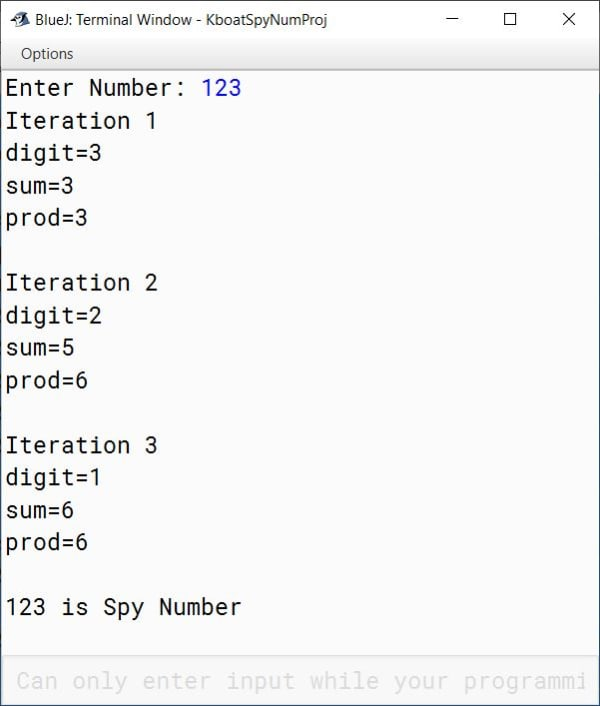# Spy Number Java Program

## What is a Spy Number?

A number is said to be a spy number if sum of its digits is equal to the product of its digits. Taking the number 1124 as an example:

Sum of digits of 1124:
= 1 + 1 + 2 + 4
= 8

Product of digits of 1124:
= 1 x 1 x 2 x 4
= 8

As the sum of digits and product of digits is equal for 1124, hence it is a Spy Number.

## Spy Number Program

This is the program to check if a given number is a Spy number or not.

```import java.util.Scanner;

public class KboatSpyNumber
{
public void spyCheck() {
Scanner in = new Scanner(System.in);

System.out.print("Enter Number: ");
int num = in.nextInt();
int orgNum = num;

int digit, sum = 0, prod = 1;

while (num > 0) {
digit = num % 10;

sum += digit;
prod *= digit;
num /= 10;
}

if (sum == prod)
System.out.println(orgNum + " is Spy Number");
else
System.out.println(orgNum + " is not Spy Number");

}
}```

Let's go over the program to understand it. In the below lines of the program:

```Scanner in = new Scanner(System.in);

System.out.print("Enter Number: ");
int num = in.nextInt();
int orgNum = num;```

we accept the number as input from the user through Scanner class and make a copy of it in variable `orgNum`.

In the line after that:

`int digit, sum = 0, prod = 1;`

we declare 3 int variables — `digit`, `sum` and `prod`. `digit` will hold the extracted digit of the number. `sum` and `prod` are used as accumulators to store the sum and product of digits, respectively.

Next we have the while loop:

```while (num > 0) {
digit = num % 10;

sum += digit;
prod *= digit;
num /= 10;
}```

This while loop will run till the number is greater than 0. Inside the loop, we extract the digit, add it to sum, compute product with it and lastly, we truncate the number.

After the loop, we have the if check:

```if (sum == prod)
System.out.println(orgNum + " is Spy Number");
else
System.out.println(orgNum + " is not Spy Number");```

In this if statement, we check if sum of digits is equal to their product and print an appropriate message accordingly.

Let us add a few print statements to get some insights into what is happening in each iteration of the loop and then we will see the output and correlate it with the code.

Here is the program with the print statements added and its output:

```import java.util.Scanner;

public class KboatSpyNumber
{
public void spyCheck() {
Scanner in = new Scanner(System.in);

System.out.print("Enter Number: ");
int num = in.nextInt();
int orgNum = num;

int digit, sum = 0, prod = 1;
int itr = 1;

while (num > 0) {
digit = num % 10;

sum += digit;
prod *= digit;
num /= 10;

/*
* for explanation
*/
System.out.println("Iteration " + itr++);
System.out.println("digit=" + digit);
System.out.println("sum=" + sum);
System.out.println("prod=" + prod);
System.out.println();
}

if (sum == prod)
System.out.println(orgNum + " is Spy Number");
else
System.out.println(orgNum + " is not Spy Number");

}
}```

#### OutputBefore the execution of while loop, value of `sum` and `prod` is 0 and 1, respectively. In the first iteration, the digit in the unit’s place which is 3 is extracted. `sum` is 0, adding digit to `sum` changes the value of `sum` to 3 as shown in the output above. `prod` is 1, multiplying it with digit makes `prod` also as 3.

Program control goes back to the beginning of while loop and tests the condition. As `num` is greater than 0, second iteration of while loop starts. Digit 2 is extracted from the number. `sum` becomes 5, `prod` becomes 6.

Again, program control goes back to the beginning of while loop. Condition is still true as number is greater than 0. Last digit of the number is extracted. `sum` becomes 6, `prod` remains 6. The number becomes 0 and third iteration of while loop completes.

Again, program control goes back to the beginning of while loop. But now the condition becomes false as `num` is 0. Program control comes to the if check after the while loop. As `sum` and `prod` are both 6, this condition tests true. 123 is spy number gets printed to the console.